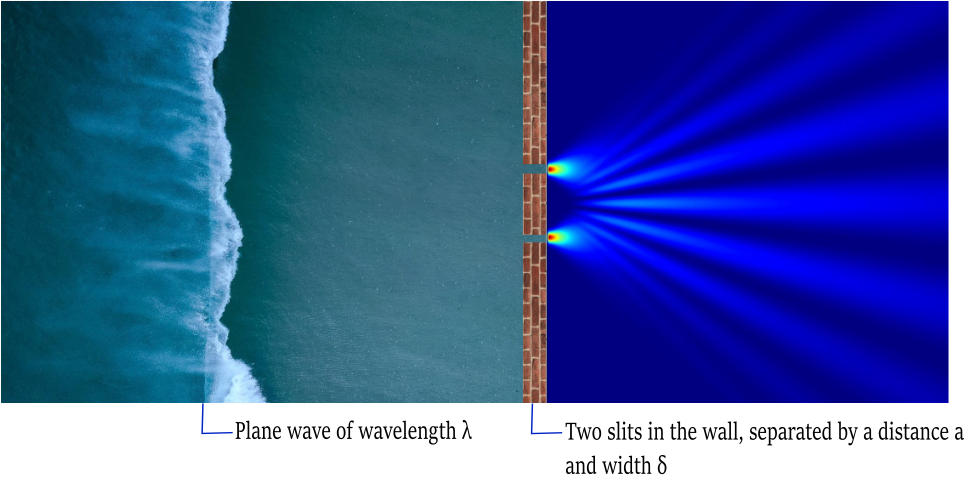### In this case we represent the standard version of Feynman's thought experiment, considering a situation where a plane wave reaches the arrangement of two slits (simulated as Gaussians of width δ separated by a distance δ / a = 0.1). After passing the slits, the wave function can be written analytically by means of two Gaussian wave packets. The image shown at the end of this section shows the probability distribution | φ (x, L) | 2 of the experiment.